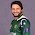## Monday, June 16, 2014

### JEE Advanced 2014 Questions on Electric Circuits

Do not worry about your problems with mathematics, I assure you mine are far greater."
Albert Einstein

The following questions appeared in  JEE Advanced 2014 question paper. Question No. 1 is a multiple choice question in which one or more than one options are correct. The second question also is multiple choice type but it has just one correct option.

(1) Heater of an electric kettle is made of a wire of length L and diameter d. It takes 4 minutes to raise the temperature of 0.5 kg water by 40 K. This heater is replaced by a new heater having two wires of the same material, each of length L and diameter 2d. The way these wires are connected is given in the options. How much time in minutes will it take to raise the temperature of the same amount of water by 40 K ?

(A) 4 if wires are in parallel

(B) 2 if wires are in series

(C) 1 if wires are in series

(D) 0.5 if wires are in parallel

The resistance R of the heater wire in the first kettle is given by

R =  ρL/A where ρ is the resistivity (specific resistance) of the wire and A is the cross section area of the wire.

[Note that A = π(d/2)2]

The second kettle has two heater wires, each of the same length L but cross section area 4A.

[Since the diameter of each wire in the second kettle is twice that of the wire in the first kettle, the cross section area is 4 times].

The resistance of each wire in the second kettle is R/4.

When the wires are connected in parallel, the effective resistance is R/8.

The power of the first kettle is V2/R where as the power of the second kettle is V2/(R/8) which is equal to 8V2/R.

Since the power of the second kettle is 8 times that of the first one, the time required for heating the water is reduced by a factor of 8 and is equal to 4/8 minutes which is 0.5 minute. Therefore (D) is a correct option.

When the wires are connected in series, the effective resistance of the combination is 2×(R/4) = R/2. The power of the second kettle is then V2/(R/2) which is equal to 2V2/R.

Since the power of the second kettle in this case twice that of the first one, the time required for heating the water is half the time taken by the first kettle and is equal to 2 minutes. Therefore (B) too is a correct option.(2) During an experiment with a metre bridge, the galvanometer shows a null point when the jockey is pressed at 40.0 cm using a standard resistance of 90 Ω, as shown in the figure. The least count of the scale used in the metre bridge is 1 mm. The unknown resistance is

(A) 60 ± 0.15 Ω

(B) 135 ± 0.56 Ω

(C) 60 ± 0.25 Ω

(D) 135 ± 0.23 Ω

We have R/90 = 40/(100 – 40) from which R = 60 Ω.

Since the least count of the scale used in the metre bridge is 1 mm only, there is an error in the value of R calculated above. In order to take this inaccuracy into account, we write the balance condition of the metre bridge as

R/90 = x/(100 x) where x is the balancing length (on the side of R as shown in the figure).

Taking logarithms,

ln R = ln 90 + ln x – ln(100 x)

Therefore R/R = x/x ∆(100 x)/(100 x)

Or, R/R = x/x + x/(100 x)

Since x = 0.1 cm, x = 40 cm and R = 60 Ω, we have

R/60 = (0.1/40) + (0.1/60)

Therefore, ∆R = 60[(0.1/40) + (0.1/60)] = 0.25 Ω

The unknown resistance is R ± R = 60 ± 0.25 Ω

#### 5 comments:

1.How is delta x =0.1cm ? 0.1cm is the least count right?

1.Anonymous12:54 PM

Its the least count of the scale.

2.Yes, indeed.

3.This comment has been removed by a blog administrator.

4.Nice try but second one is not fully explained